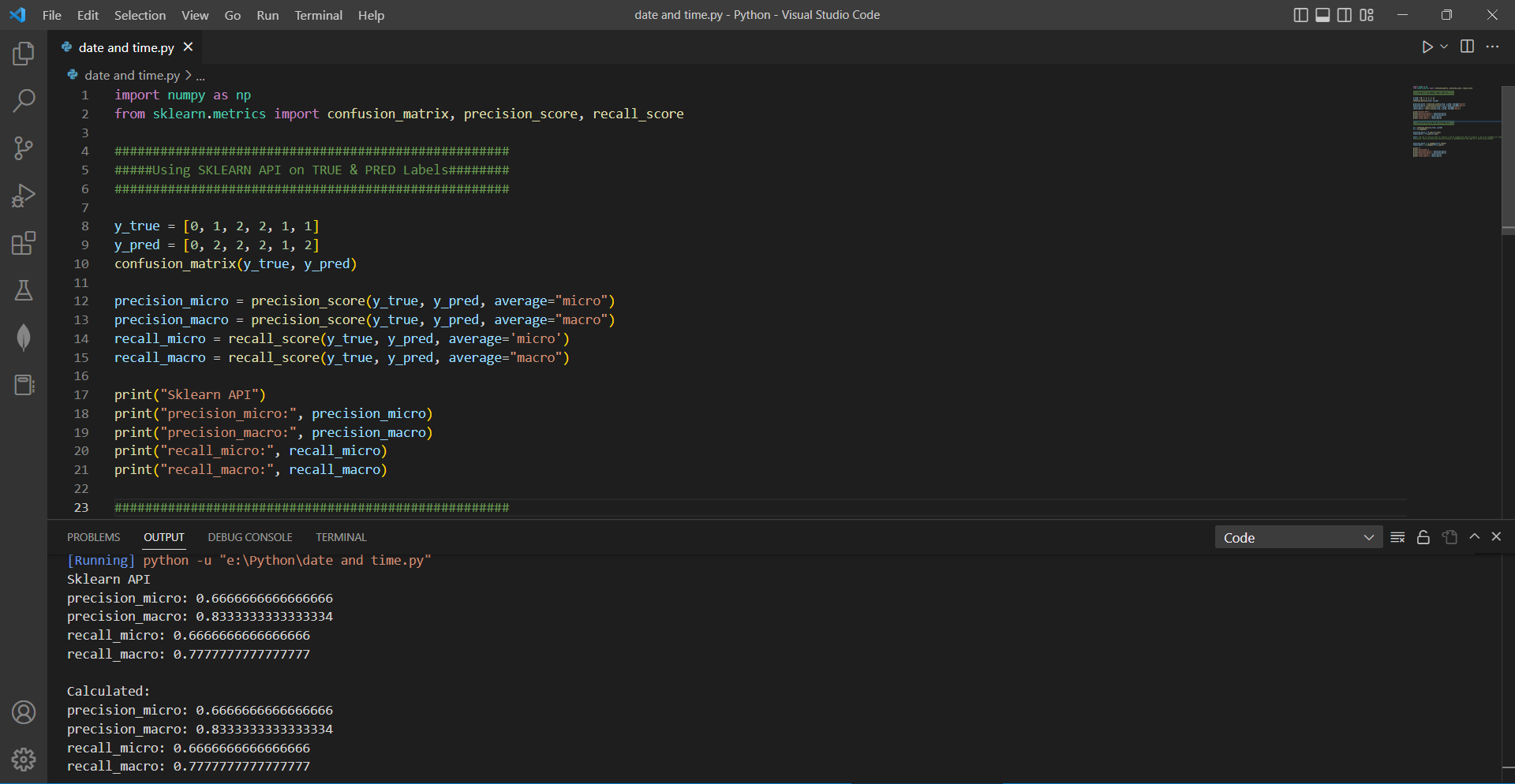# Finding the precision and recall for a confusion matrixby vigneshchennai74Updated: Feb 16, 2023Solution Kit

Precision and recall are two commonly used metrics for evaluating the performance of a classification model. Precision measures the accuracy of the positive predictions, while recall measures the ability of the model to identify all relevant positive samples. y_true is the list of true labels and y_pred is the list of predicted labels. The precision_score and recall_score functions calculate the precision and recall, respectively

Precision is the fraction of true positive predictions out of all positive predictions made. It Measures the accuracy of the positive predictions

recall is the fraction of true positive predictions out of all actual positive cases. It measures the completeness of the positive predictions

• Confusion_matrix: This function generates a confusion matrix given true labels and predicted labels.
• precision_score: This function calculates the precision score of a classification model given true labels and predicted labels.
• recall_score: This function calculates the recall score of a classification model given true labels and predicted labels.
• These libraries and functions can be used to evaluate the performance of a classification model.

Here is the example of how we can find the Precision score and recall score using Sk-learn.Preview of the output that you will get on running this code from your IDE﻿

### Code

In this solution we have used Scikit-Learn

1. Copy the code using the "Copy" button above, and paste it in a Python file in your IDE.
2. Run the file to get the output

I hope you found this useful. I have added the link to dependent libraries, version information in the following sections.

I found this code snippet by searching for "Need help finding the precision and recall for a confusion matrix" in kandi. You can try any such use case!

### Dependent Library

scikit-learnby scikit-learn

Python54382Version:1.2.2scikit-learn: machine learning in Python

Support
Quality
Security
Reuse

scikit-learnby scikit-learn

Python54382Version:1.2.2License: Permissive (BSD-3-Clause)

scikit-learn: machine learning in Python
Support
Quality
Security
Reuse

numpyby numpy

Python23587Version:v1.24.3The fundamental package for scientific computing with Python.

Support
Quality
Security
Reuse

numpyby numpy

Python23587Version:v1.24.3License: Permissive (BSD-3-Clause)

The fundamental package for scientific computing with Python.
Support
Quality
Security
Reuse

### Environment Tested

I tested this solution in the following versions. Be mindful of changes when working with other versions.

1. The solution is created in Python 3.7.15 version
2. The solution is tested on scikit-learn 1.0.2 version

Using this solution, we are able going to learn how to Finding the precision and recall for a confusion matrix in python using Scikit learn library in Python with simple steps. This process also facilities an easy to use, hassle free method to create a hands-on working version of code which would help Finding the precision and recall for a confusion matrix in Python.

If you do not have Scikit-learn and numpy that is required to run this code, you can install it by clicking on the above link and copying the pip Install command from the Scikit-learn page in kandi.

You can search for any dependent library on kandi like Scikit-learn. numpy

### Support

1. For any support on kandi solution kits, please use the chat
2. For further learning resources, visit the Open Weaver Community learning page.

See similar Kits and Libraries